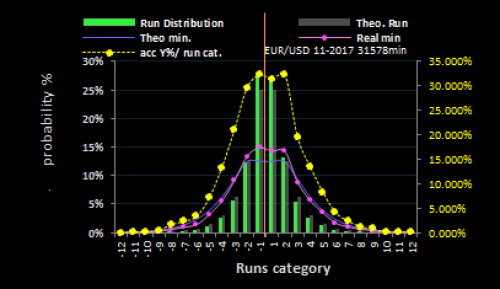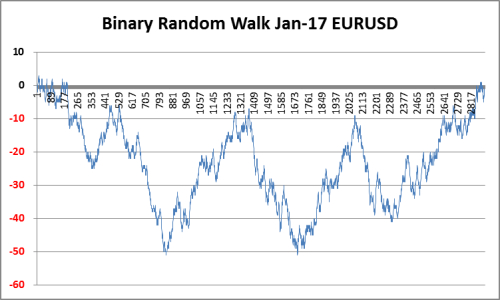Welcome and background
1500 words are enough to tell you "that I love you", but to show randomness in the currency market with those words is a though test of restricting your writing, but I will try.

I have spend more than 2 years in research on randomness in financial markets. I was frustrated to have this invisible and disputed partner of randomness running around in disguise, in my price chart. I finally found this partner and it proved to be a friend instead of an enemy, you can use the randomness to your trading advantage, so do not fear it.

Terms
The time period I use for one move is one minute. The currency is EURUSD.

A move is either up or down. 1 denotes up and -1 denotes down. A move = 1 trading minute.

A run is a count of the consecutive times the price moves in only one direction. Runs can thus be up (positive) and down(negative). A "short" must perceive down (negative) in the context of my article and not as a trade.

Yield is defined as the difference between the next price and the present price.

Assumptions to be verified
IF there are traces of Randomness in the market, what might we expect to find, that could verify it's presence ?

a) If I accumulate all positive moves in one box and all negative moves in another and sum them up I will have the total moves.
(Positive moves / All moves)= Pmr (positive move ratio) and if randomness is present, the Pmr ratio should be close to 50%. Why? Because a random model with 2 outcomes has 50% probability for up and 50% probability for down.

b) If I do the same with the yield I will get a Pyr (positive yield ratio) and if I assume it is random I expect Pyr to be app. 50%. Why ? Because if the yield is equally distributed on positive and negative Pyr will be app. 50%.

c) A frequency distribution of runs and accumulated minutes per run should follow the theoretic random distribution of runs and minutes, if runs in currencies are randomly distributed. (Coin flips have such a run distribution.)

Oscillation / drift away from the perfect balance in a random system
When Randomness unfolds it's nature in reality, it never follows the theoretic distribution to the last decimal, it is drifting away from the 50% mark to one of the sides. Another way to say it is the difference between positive and negative ratios (50%-50%) is seldom zero. So Pmr and Pyr will not show precisely 50%, but will be a bit over or under 50%. "A bit" depends on the length of the period you analyze ( the shorter the period, the more is "a bit" and opposite), but to have something to refer to let us say from 49%-51%.

Pmr and Pyr ratios
Then we are ready to take a look of how the assumptions compare to the reality and we start with points a and b. The period is 2014-2017 for EURUSD based on minute ticks and calculated on monthly basis. Left chart is Pmr and right chart is Pyr.Both Pmr and Pyr range mostly between 49.40% and 50.40% and drift over the 50% middle, with a few outside that range. Each point has its own "allowance" for the drift, but this article does not allow space to detail it. But 49-51% is a suitable rough estimate. It does not mean that results outside that range are not random, but it means the probability for it to happen is very low.

The average of all 4 years for Pmr is 49.95% and Pyr 49.98% That is close to 50-50 and pmr has a "drift range" over 4 years of 49.93% to 50.12%.

Our assumptions from point a and b are strongly verified, the EURUSD currency pair have random behavior.

Run and minute frequency distribution versus the theoretic distributions. (refers to c.)
EURUSD Nov-2017Green bars are the real data distribution of runs and grey shaded is the theoretic distribution of runs. The pink is the distribution of trading minutes over the runs. The blue line is the theoretic distribution of minutes over the runs. (read all results on left vertical axis)

The yellow has no theoretic distribution, because it is the accumulated Yield % distributed over the runs. Read results on the right vertical axis.

The orange line is the mid point of the distribution. Negative runs are to the left and positive runs are to the right. Runs are just a classification of the trading minutes, so the run distribution(green/grey)can be calculated into it's corresponding trading minute distribution.(pink/blue)

The same rule applies here, that shorter periods allow for more drift from the theoretic distribution than longer periods. They are all close to the theoretic distribution. About 50% of all runs are +/- 1 and about 25% are +/- 2, so app. 75% of all runs are +/- 1 or 2 and the minutes they cover is app. 50% of all trading minutes. The percentage on the right vertical axis tell us, that run of -1 accumulate about 32% negative yield and +1 a bit less, so the Yield contribution to the total yield from runs +/-1 is negative, but for 2 it is positive.
You cannot see it in the graphic, but the Pmr was 50.51% and the Pyr was 50.53% and the negative side was thus (100%-50.51%)= 49.49% and (100%-50.53%) = 49.47%.

It "seems" there may be "some relation" between the pink minute distribution and the yield distribution and it would be really nice, if we were able to determine the yield, by having the minute distribution. It is not as easy as it may seem by glance, but it is also not impossible to get some qualified estimates out of this discovery, but it would be an article in itself to dig deeper into that subject.

However our assumption in c is confirmed, the runs and trading minutes distribute themselves in accordance with the random distributions.

The theoretic probabilities in trading can be described with the graph below and be a reminder to a trader of what "normally" happens in trading in the long run and why you can get stressed, annoyed and feel the higher powers in the market simply have pin pointed you, just to give you bad luck.

The theoretic accumulated probability curve of runs and minutes in a trading session.It requires about 1.000 trading minutes to expect a run of 10 to either side. The bars around the run number on the x axis means "absolute", so it covers both run 1 and -1 a.s.o. C means accumulated. Thus the probability for run -1 is 25% and for run +1 25% and for absolute 1 (25%+25%)=50%

50% of all runs are absolute 1 and they cover 25% of all trading minutes. If we include runs of 2, they cover 75% of all runs and 50% of all minutes. Small runs can improve a trade, if there are enough in the right direction, but it can take long time and it is more rewarding, to get a run of 8 which benefits the trade. But they do not come often, 93.75 of all runs are expected to be within 1-4 runs and 81.25% of all minutes are traded within these runs. It means the really good runs from 5-10 with some attractive yield comes in app. (99.41%-81.25%) = 18.16% of the minutes.

Random WalkA Random walk in currencies goes only from left to right and up or down. Some articles makes mistakes about it and give them right to left move as well, that is wrong.

Binary means it is counted in moves. There were net negative moves in the period and drifting to and back and returned to zero in app. 3.000 minutes.

This article made randomness visible and it was chased out of the price chart. It showed a strong verification of randomness in currencies. We can only verify it, but not prove it.
Translate to English Show original